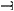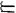# Ordered and Partially Ordered Sets

The following article is from The Great Soviet Encyclopedia (1979). It might be outdated or ideologically biased.

## Ordered and Partially Ordered Sets

in mathematics, sets with a binary relation of order or partial order. A relationpartially orders a set M if some pairs of elements in M are related byand (1) xx is false for all xM and (2) xy and yz imply xz (transitivity of). If for all pairs of different elements x, y in M we have xy or yx, then we say thatorders M. If xy, then it is customary to say that x precedes y and that y follows x. For example, by saying that the complex number a′ + bi follows the complex number a + bi provided a′ > a and b′ > b, we partially order every set of complex numbers and, in particular, every set of real numbers. Since in this case a real number a′ follows a real number a if and only if a′ is greater than a, our definition orders every set of reals. The concepts of partial order and order are among the basic general concepts of mathematics (seeSET THEORY).

Well-ordered sets. An ordered set is said to be well-ordered if each of its subsets contains a first element, that is, an element that precedes all other elements. Ordered finite sets and the natural numbers ordered by magnitude—as well as in some other ways—are examples of well-ordered sets. The main reason for the importance of well-ordered sets is that the principle of transfinite induction applies to such sets.

Ordered sets of the same order type have the same cardinality, and we can therefore speak of the cardinal number of an order type. Finite ordered sets of the same cardinality are of the same order type. This need not be true of infinite sets; that is, infinite ordered sets may have different order types.

Directed sets. Define ab to mean that ab or a = b. A partially ordered set is said to be directed if for every two of its elements x and y there exists an element z such that zx and zy. The concept of a directed set enables us to give a very general definition of limit. For simplicity, let f(p) be a number-valued function defined on a directed set M. A number c is called the limit of f(p) with respect to the directed set M if for every ∊ > 0 there exists a p̄ ∊ M such that for all pM with pp̄, ǀf(p) – cǀ < ∊. This concept has all the usual properties of limit and covers a very large number of special cases not covered by the usual notion of limit.

Historical note. The theory of ordered sets is due to G. Cantor. In 1883, Cantor introduced the concept of a well-ordered set and in 1895 the concepts of ordered set and order type. In 1906–07, S. O. Shatunovskii formulated the definitions of directed set and limit with respect to a directed set. These concepts were independently discovered by the American mathematicians E. H. Moore and J. L. Smith in 1922. The general concept of a partially ordered set was introduced by F. Hausdorff in 1914.

### REFERENCES

Aleksandrov, P. S. Vvedenie v obshchuiu teoriiu mnozhestv i funktsii. Moscow-Leningrad, 1948.
Kurosh, A. G. Lektsii po obshchei algebre, 2nd ed. Moscow, 1973.
Hausdorff, F. Teoriia mnozhestv. Moscow-Leningrad, 1937. (Translated from German.)
Kuratowski, K., and A. Mostowski. Teoriia mnozhestv. Moscow, 1970. (Translated from English.)
Bourbaki, N. Teoriia mnozhestv. Moscow, 1965. (Translated from French.)